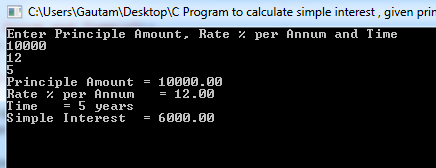# C Program to Calculate Simple Interest by Given Principle, Rate of Interest and Time

Simple interest is calculated by, sinterest=principle*time*rate/100 formula.

Program:
``````#include <stdio.h>

int main()
{
float principle, rate, sinterest;
int time;

printf("Enter Principle Amount, Rate %% per Annum and Time\n");
scanf ("%f %f %d", &principle, &rate, &time);

sinterest = (principle * rate * time)/ 100.0;

printf ("Principle Amount = %5.2f\n", principle);
printf ("Rate %% per Annum   = %5.2f%\n", rate);
printf ("Time   = %d years\n", time);
printf ("Simple Interest  = %5.2f\n", sinterest);
}``````
Program Output:Explanation:

This program will calculate the value of the SI where the principal, rate and the time is given by the user. So first of all, you have to include the stdio header file using the "include" preceding # which tells that the header file needs to be process before compilation, hence named preprocessor directive. There is also another header file conio.h, which deals with console input/output and this library is used here for getch() function.

Then you have to define the main() function and it has been declared as an integer so by default it returns the integer. Inside the main() function you have to declare floating type variables name 'principle', 'rate', 'sinterest'; Moreover, you have to take another integer type variable name 'time'. Now, use printf() to display the message: "Enter Principle Amount, Rate %% per Annum and Time". Then the scanf() method is used which will take three values from the user and store it into variables - principal, rate and time.

Then according to the formula of simple inerest, the multiplicative value of all three i.e. (principal * rate * time) gets divided with 100.0 and is stored in the variable 'sinterest'.

Then all the four values i.e. principal, rate and time along with the calculated value of simple interest gets displayed using four printf() statements. The getch() reads a single byte character from input and getch() is a proper way to fetch a user inputted character.